## Can this Jacobi Differential equation be solved?...

I have been trying to find a solution for the equation below. Is there a non numerical explicit solution?(1)(2)(3)(4)(5)(6)(7)(8)(9)(10)(11)## How to find equation of the intersection between ...

With the following command I can plot two spheres and plot them.

f1 := x^2+y^2+z^2 = 1

f2 := x+y+z = 1

with(plottools);

with(plots);

S1 := implicitplot3d(f1, x = -1 .. 1, y = -1 .. 1, z = -1 .. 1, style = patchnogrid, color = blue, scaling = constrained, axes = boxed)

S2 := implicitplot3d(f2, x = -1 .. 1, y = -1 .. 1, z = -1 .. 1, style = patchnogrid, color = gold, scaling = constrained, axes = boxed)

dispaly(S1,S2)

My questions are:

1- How can I display (highlight) the circle which is the intersection between these two sphere on the same figure?

2- How can I find the equation of this circle?

Thank you.

## What is the simplest way to assign the values of ...

Hi,

I have two same dimentional matrix(1000*1000), one is full of variables and the other is full of numbers. What is the simplest way to assign the values of second matrix to first one variables?

for example:

A := Matrix([[x, y], [z, t]]);

B := Matrix([[1, 2], [3, 4]]);

How assign B's values to A's variables, in such a way that after assigning ,when call x, the 1 appears in output.>>(1)
 >(2)
 >>(3)Is it possible get a solution of this equation without RoofOf in form explicit?## Undoing the effect of kronecker delta functions...

I am solving a matrix eigenvalue problem, and have found a way to make the matrices very sparse by a set of conditions x=x', y=y' and z=z'. If these are satisfied then the matrix element will be >0. If any one of them is not satisfied, then the matrix element becomes zero; hence a sparse matrix is formed. Mathematically in Maple this can be represented as such:

ME*KroneckerDelta[x,xp]*KroneckerDelta[y,yp]*KroneckerDelta[z,zp];

Where ME represents the matrix element and xp, yp, zp represent the prime notation . I apply this form within a C++ routine which I am extracting the matrices from into Maple where I am looking to modify them to experiment with an extension of the program.

The matrices I read into Maple have had the Kronecker delta functions applied, however Is there a simple way to reverse the effect of the Kronecker delta functions with the Matrix in Maple and return the Matrix equivalent to just ME without the product of kronecker delta functions?

-Yeti

## Include a maple file in another...

In the same directory I have two Maple files. SignExpAlg.mw and RootBox.mw

In SignExpAlg.mw I have written several procedures which I am wanting to use in RootBox.mw

I have tried to do

at the top of RootBox but when I click !!! I get the error message: "Error, could not open 'SignExpAlg.m' for reading"

What am I doing wrong?

## What are these notations?...

I don' t understand what do these notations stand for? I know that there are some notations like these in Maple. How can I find meanings of the all of them?## Strange behavior of t-distribution command in Mapl...

I am finding that the  PDF command with Student's t-distribution in the Statistics package is not behaving as expected. Here is what I tried so far:

>restart;
>with(Statistics):
>X := RandomVariable(StudentT(nu));

>PDF(X,0.5);
Dirac(X - 0.5)

Note that PDF(X,0.5) is evaluating to Dirac(X-0.5) instead of the pdf of Student's t-distribution density function.

Any help in identifying the issue is greatly appreciated. I am running Maple2015 on linuxmint 17.

Thanks!

## How do I speed up the Evaluation of Elliptical fun...

Question:- can the procedure given below called "epi" be speeded up by compiling/ using evhf.If so how? My paple code is at the bottom.

First some background information.

Recently I ran into a difference in usage of a couple of elliptical functions between Maple and Mathematica.  This first case concerned EllipticalPi. The author of the blog kindly wrote  a Maple procedure to produce the same results as Mathematica’s  usage of ElllipticalPi.

I tested the basic integral that produces the EllipticPi    Ell := int(1/(1-nu*JacobiSN(t, k)^2), t)  answer      Ell := EllipticPi(JacobiSN(t, k), nu, k). They do not produce the same outcome. Plots are in the document .  They agree in one quarter only.

I then ran into a difference in usage of  EllipticF. This time I was able to get to same outcome myself using Maple’s help.

“It is worth noting the difference between the Legendre normal form of the Incomplete Elliptic integral of the first kind (see A&S 17.2.7), in Maple represented by EllipticF(z,k) but for the splitting of the square root in the denominator of the integrand (see definition lines above), and the normal trigonometric form of this elliptic integral (see A&S 17.2.6), in Maple represented by the InverseJacobiAM function
InverseJacobiAM(phi,k);

That worked fine.

There is no mention in the help for usage implementation of EllipticPi as opposed to different usages as there is with EllipticF. I do not know if there is a way in Maple of achieving the same enactment as Mathematica in this case, without the Procedure I  was  given.

Elliptic Pi in Mathematica and Maple

We use EllipticPi when we write exact solutions of rotation of a free asymmetric top. While solving Euler’s equations for angular velocity or angular momentum in the body frame we need Jacobi elliptic functions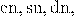solving the differential equation for the attitude matrix involves EllipticPi function. As I have explained it in Taming the T-handle continued we need the integral

(1)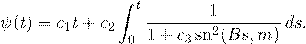In Mathematica this is easily implemented as

(2)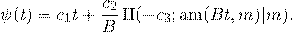However, as pointed out by Rowan in a comment to Taming the T-handle continued , the same formula does not work with Maple.

While the documentations of both Mathematica and Maple contain links to Abramowitz and Stegun Handbook of Mathematical Functions, they use different definitions. Here is what concerns us, from p. 590 of the 10th printing: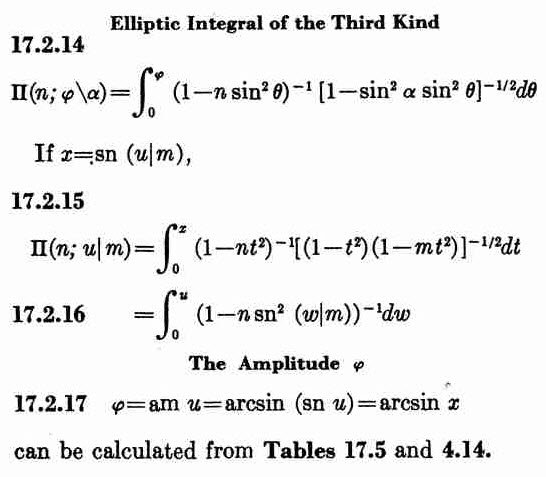What we need is 17.2.16, while Maple is using 17.2.14. To convert we need to set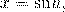but such a conversion is possible only in the domain where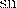can be inverted. We can do it easily for sufficiently small values of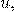but not necessarily for values that contain several quarter-periods.

The following Maple procedure does the job:

epi := proc (t::float, nu::float, k::float) local t2, n, dt, ep0, res; ep0 := EllipticPi(nu, k); t2 := EllipticK(k); n := floor(t/t2); dt := t-t2*n; if type(n, even) then res := Re(n*ep0+EllipticPi(JacobiSN(dt, k), nu, k)) else res := Re((1+n)*ep0-EllipticPi(JacobiSN(t2-dt, k), nu, k)) end if end proc

HAs an example here is the Maple plot for nu=-3, k=0.9:
plot(('epi')(t, -3.0, .9), t = -20 .. 20)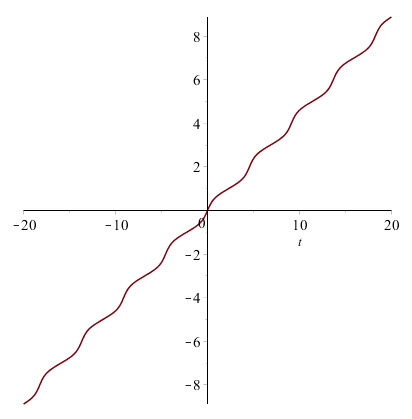And here is the corresponding Mathematica plot: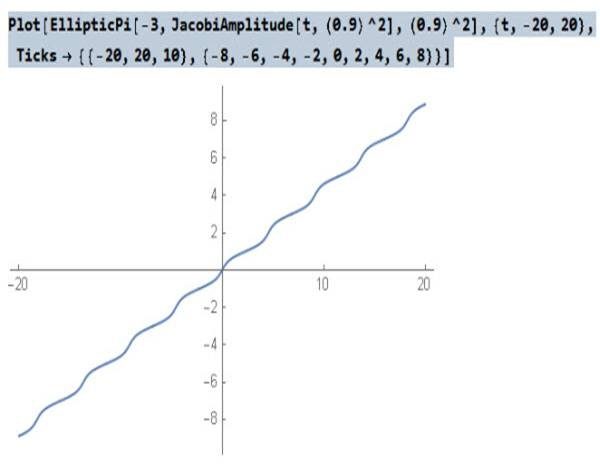The function epi(t,nu, k) defined above for Maple gives now the same result as EllipticPi(nu,JacobiAM(t,k^2),k^2) in Mathematica.

restart;
epi := proc (t, nu, k) local t2, n, dt, ep0, res; ep0 := EllipticPi(nu, k); t2 := EllipticK(k); n := floor(t/t2); dt := t-t2*n; if type(n, even) then res := Re(n*ep0+EllipticPi(JacobiSN(dt, k), nu, k)) else res := Re((1+n)*ep0-EllipticPi(JacobiSN(t2-dt, k), nu, k)) end if end proc;

Ell := int(1/(1-nu*JacobiSN(t, k)^2), t);
Ell := EllipticPi(JacobiSN(t, k), nu, k)
k := .9;
k := 0.9
nu := -3;
nu := -3
plot([epi(t, nu, k), Ell], t = -8 .. 20);

## MapleTA graphing algorithm...

Hi all,

I am attempting to construct a randomized questions using algorithms and the sketch question type.

I have defined my variables algorithmically.  The sketch question type only allows a static answer, and hence I am trying to modify the code.

As you can see below the code (part.1.answer...) is looking for points located at (7,0) and (0,-7).  I would like to replace the 7 with variable $xint and -7 with variable$yint, but it seems the @-symbols are some form of termination point that won't allow variables to carry-into the expression.

Looking for ideas??  I do not want to change to multiple choice.

Thanks,

mode=Inline@
id=0@
name=Graph a linear relation in Ax+By=C form@
comment=<p>&nbsp;</p>

@
editing=useHTML@
solution=@
algorithm=$b=range(1,6,2);$c=range($b,20,$b);
$a=$b*3;
$zero=0;$xint=$c/$a;
$yint=$c/$b;@ customCss=@ appliedThemes=@ uid=316f7dda-d8bc-479f-a3e4-9703a1846072@ privacy=10@ allowRepublish=false@ description=@ difficulty=0.0@ modifiedIn=11.10@ modifiedBy=a0c70480-e1aa-418d-9980-0347cddc1087@ school=7f01c0fb-116a-4f9d-bafc-e91de0b62105@ attributeAuthor=true@ numberOfAttempts=1@ numberOfAttemptsLeft=1@ numberOfTryAnother=0@ numberOfTryAnotherLeft=0@ question=<p><span style="font-size:16px;">Graph &nbsp;<math xmlns="http://www.w3.org/1998/Math/MathML"><mrow> <mn>$a</mn> <mspace width="5px"></mspace> <mi mathvariant="italic">x</mi> <mo>+</mo> <mi mathvariant="italic">&nbsp;</mi> <mn>$b</mn> <mspace width="5px"></mspace> <mi mathvariant="italic">y&nbsp;</mi> <mo>=</mo> <mn>$c</mn> </mrow>[/itex]&nbsp; on the grid below.</span></p>

<p>&nbsp;</p>

<p><span>&nbsp;</span></p>

<1>

<p><span>&nbsp;</span>&nbsp;</p>

@
weighting=1@
numbering=alpha@
part.1.grid=true@
part.1.comment=<p>&nbsp;</p>

@
part.1.editing=useHTML@
part.1.attributeAuthor=false@
part.1.options="visible_buttons":"createline:1, delete"@
part.1.modifiedIn=11.10@
part.1.numberOfAttempts=1@
part.1.allowRepublish=false@
part.1.numberOfTryAnotherLeft=0@
part.1.numberOfAttemptsLeft=1@
part.1.mode=Html5sketch@
part.1.numberOfTryAnother=0@
part.1.gradingCode=op(1,[Grading:-GradePlot($ANSWER,$RESPONSE, \$PLOTVIEW)]);@
part.1.axes=-10,10,-10,10@
part.1.difficulty=0.0@
part.1.ticks=4,5,4,5@
part.1.name=responseNaN@

## Maple to adjust size of plot output by right click...

I am trying to find a way to adjust the size of a plot output component in Maple by right click (because the output(s) were copy & paste) and it is difficult to go back to plot command to set the size.

Thanks,

Dear All,

This is probably an easy issue to solve.

I have imported an .xls file using the 1D lookup table.

I need the signal to be executed for 4 seconds which is also how long my simulation duration is.

So I set the Ramp height and rise time to 4s. For some reason when I display the signal using a probe it gives me the constant value of the first row of my Excel sheet. I need help to solve this! I have provided some visuals below.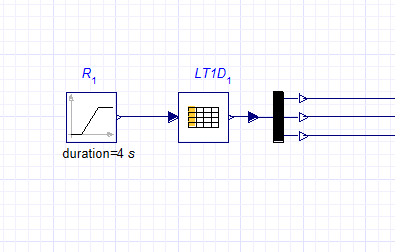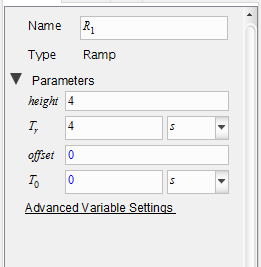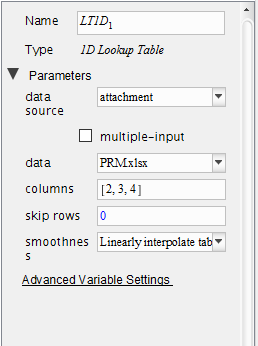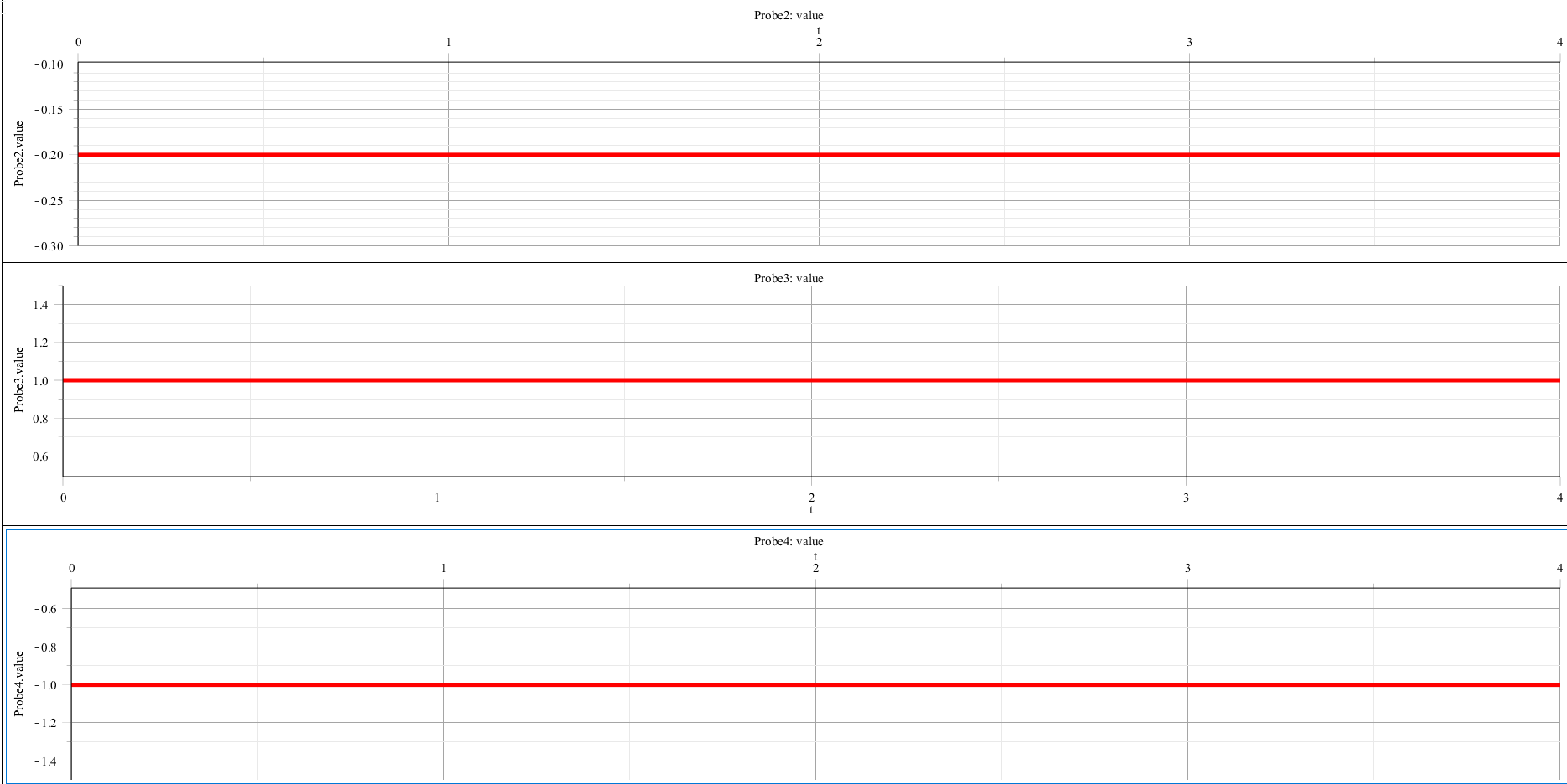Can

## Getting de magnitude of a complex number...

I don't know why my code don't work, I'm trying to calculate the magnitude of a complex vector but I get a error in the next image you can view it,Can someone help me? thank you

## How is it possible the maple do not open those sub...

My program have more than 100 subsections. It is tedious to close them after run.

How is it possible the maple do not open those subsections I specified, during running the program?

Is it possible to close subsections abruptly?

## Function with both explicit and implicit dependenc...

Greetings People!

I am modelling a dynamical system using variational formulation. The final step is to plug in, the energy values, in the Euler-Lagrange Equation. The functional has both explicit dependence on time, and implicit dependence (in the form of the generalised coordinate). Since motive of the exercise is to obtain the governing equations for the generalized coordinates, this implicit dependence is unknown. How can Maple be used to derive the differential equations in this situation?

As an example, consider,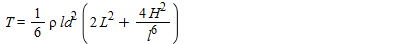Here, ld and l are variables, which are function of time. In order to calculate the term,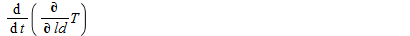How do I proceed? Thanks in advance.

﻿